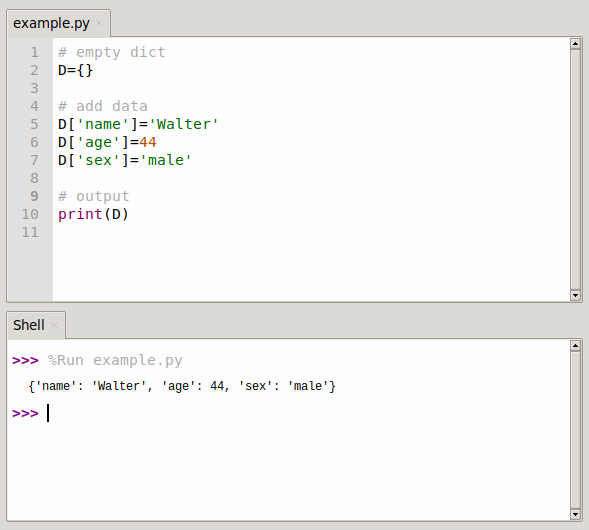# Create a dictionary in Python

There are many ways to create a dictionary, and depending on the needs, the right way to do it is the right way to do it with half the effort.

I’m using Python 3.0, so a new parsing creation method has been added compared to the 2.X version.

A dictionary is an unordered set, a key > value mapping. Each key maps to exactly one value.

## Create dictionary in Python

I call this method a bracketing method, which is suitable for you to know what’s already in the dictionary and create the dictionary.

``````"""
1. One curly brace for the whole method
"""

D={'name':'Bob','age':14,'sex':'male'}
print(D)``````

Output.

``{'name': 'Bob', 'age': 14, 'sex': 'male'}``

## Empty dictionary

When faced with the situation of adding fields to the dictionary again and again, we first create an empty dictionary and then fill in the contents.

Shows fields dynamically created in the dictionary

``````D={}
D['name']='Bob'
D['age']=14
D['sex']='male'
print(D)``````

Output.

``{'name': 'Bob', 'age': 14, 'sex': 'male'}``## dict(key1=value1,key1=value2,…)

This method is easy to use and simple to use with a small amount of code~

``````"""
3. dict(key1=value1,...) equal sign form
"""
D=dict(name='Bob',age=14,sex='male')
print(D)``````

Output.

``{'name': 'Bob', 'age': 14, 'sex': 'male'}``

## dict([(key1,value1),(key2,value2),…])

Use the form (key, value) pair to create a mapping relationship object.
If you need to run the program again, gradually build the keys and values into a sequence.

This method is also often used in conjunction with the zip function, which combines different lists of keys and values that are dynamically acquired while the program is running.

``````"""
4.dict([(key1,value1),(key2,value2),...])
"""
D=dict([("name", "Bob"), ("age", 14), ("sex", 'male')])
print(D)``````

Output:

``{'name': 'Bob', 'age': 14, 'sex': 'male'}``

### How to use the combined zip function

``````D=dict(zip(['name','age','sex'], ['Bob',14,'male']))
print("zip way:",D)``````

output

``zip way: {'name': 'Bob', 'age': 14, 'sex': 'male'}``

## dict.fromkeys(key[,default])

Create a new dictionary based on the key value and value provided by the seq.
If the value is not given, the default is none.

If all the keys of the created dictionary have the same value, simply pass a list of keys, and all the keys correspond to the initial value.

``````"""
5.dict.fromkeys(key[,default])
If all the keys of the created dictionary have the same value, simply pass a list of keys, and all the keys correspond to the initial value.
"""
key_list=['name','age','sex']
D=dict.fromkeys(key_list,0)
print("keylist:",key_list)
print("Dictionary:",D)``````

Output:

``````keylist: ['name', 'age', 'sex']
Dictionary: {'name': 0, 'age': 0, 'sex': 0}``````

## dict(iterator) dictionary parsing expressions

In Python 3.0, one big difference from the previous version 2.X is the creation of dictionaries that support parsing expressions.

In this way, the dict() constructor is called, passing in an iterator object.

``````D={k:v for (k,v) in zip(['name','age','sex'],['Bob',14,'male'])}
print(D)``````

Output:

``{'name': 'Bob', 'age': 14, 'sex': 'male'}``

This approach achieves the same goal as using the zip() function directly, but looks more cumbersome than the zip function.

In fact, parsing expressions uses more scenarios than zip functions, which are more flexible and convenient.

## Dictionary examples

A whole host of examples are coming.

Example 1.

``````D={k:k **2 for k in [1,2,3,4]}
print(D)``````

Example 2.

``````D={c:c * 4 for c in 'SPAM'}
print(D)``````

output

``{'S': 'SSSSS', 'P': 'PPPP', 'A': 'AAAA', 'M': 'MMMM'}``

Example 3.

``````'''
There are also functions that can be used in conjunction with
'''

D={k.lower():k+'!' for k in 'HAPPY' }
print(D)``````

output

``{'h': 'H!', 'a': 'A!', 'p': 'P!', 'y': 'Y!'}``

Example 4: Can serve the purpose of initializing value

``````'''
To achieve the same effect as fromkeys.
'''
D={k: "initial value" for k in ['sex','name','age']}
print(D)``````

output

``{'sex': 'initial value', 'name': 'initial value', 'age': 'initial value'}``

## Dictionary Explanation.

Dictionary parsing can also include forms such as nested loops, and if words, etc.